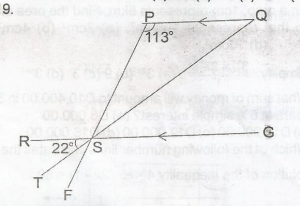In the diagram, O is the centre of the circle, < XOZ = (10cm)o and < XWZ = mo. Calculate the value of m.

A. 30°
B. 36°
C. 40°
D. 72°

Explanation

In the diagram above, α = 2m° (angle at centre = 2 x angle at circumference)

α + 10m° = 360° (angle at circumference)

α + 10m° = 360° (angles round a point)

2m° + 10m° = 360°

12m° = 360°

m° = 360°/12

= 30°